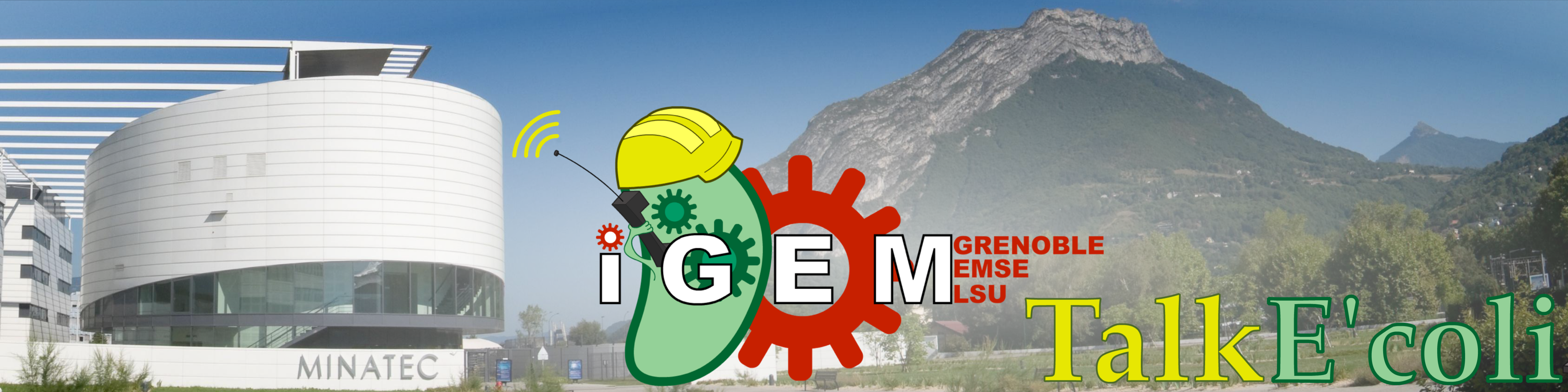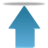# Team:Grenoble-EMSE-LSU/Project/Modelling/Model

Grenoble-EMSE-LSU, iGEMGrenoble-EMSE-LSU, iGEM

• # Modeling

Modeling took a large place in the project; it was not only used for the characterization of KillerRed and the Voigt plasmids, it was needed for the control of the bacteria’s population. With our device, we cannot control a population of living cells with a simple closed-loop transfer function. First, this is because optical measurements (OD 600 nm or fluorescence) originate from all cells, whether they are alive or not. This fluorescence intensity gives clues about living cell activity and therefore its temporal evolution permits to find the number of living cells, but there is no simple relation between them. Second, there is a large delay between an action and its effect: there are about one or two hours between the onset of illumination and the deceleration of fluorescence. In those conditions, a simple closed-loop transfer function is predictably unstable, and a model predictive control is needed to stabilize the population of living cells.

• # Building the Model

## Initial Model

### The equation

Our system is made of bacterial cells and ‘KillerRed’ proteins. Bacteria divide and produce KillerRed proteins, and KillerRed proteins respond to light: they fluoresce, degrade (photobleaching) and produce Radical Oxygen Species or ROS (phototoxicity). These reactions are exhibited by all fluorescent proteins, but the 3D structure of KillerRed makes its degradation quicker and its high concentration allows ROS to reach proteins, DNA and membrane within the bacteria and damage its vital functions. )

$\bullet$ $C$ the amount of living bacteria per milliter of cell suspension.

$\bullet$ $K$ the amount of KillerRed inside the living bacteria per milliter of cell suspension.

$\bullet$ $I$ the amount of incident (white) light.

The evolution of C and K is linked to I by the set of equations :

$\left\{ \begin{array}{l l} \frac{dC}{dt}=rC-kIK \\ \frac{dK}{dt}=aC-bIK-kI\frac{K^2}{C} \\ \end{array} \right.$

$\diamond$ $rC$ describes bacterial growth.

$\diamond$ $kIK=kI\frac{K}{C}C$ the amount of bacteria killed by KillerRed and light.

$\diamond$ $aC$ the production of KillerRed.

$\diamond$ $bIK$ the amount of KillerRed photobleached.

$\diamond$ $kIK\frac{K}{C}$ the amount of KillerRed in the bacteria killed in the last step of time.

Unfortunately, $C$ and $K$ are not measurable variables. The only thing we can quickly and easily measure are the optical density (OD) bound to the amount of bacteria dead AND alive, and the global fluoresence bound to the amount of KillerRed in the bacteria dead AND alive. To be able to compare our model to experimental results, we need two other variables :

$\bullet$ $D$ the amount of dead bacteria

$\bullet$ $K_D$ the amount of KillerRed inside the dead bacteria

$\left\{ \begin{array}{l l} \frac{dD}{dt}=kIK \\ \frac{dK_D}{dt}=kI\frac{K^2}{C}-bIK_D\\ \end{array} \right.$

The simplest possible units were used, that correspond to the measurable quantities :

$C$ and $D$ are in '$OD_600nm$' units.

$K$ and $K_D$ are in 'units of fluorescence' : UF. Bacterial auto-fluorescence is considered as negligible compared to KillerRed fluorescence.

### Analytical Solution

This simple model can be partially solved, for $C(t)$ or $I(t)$ constant for example :

If we $C$ is constant, $\forall t, C(t)=C_0$, we have :

$\left\{ \begin{array}{l l} rC_0=kIK \\ \frac{dK}{dt}=aC_0-bIK-kI\frac{K^2}{C_0}\\ \end{array} \right.$

and so : $\frac{dK}{dt}=\left(a-\frac{br}{k}\right)C_0-rK$

which gives : $K=\left(\frac{a}{r}-\frac{b}{k}\right)C_0+Be^{-rt}$

then $I(t)=\frac{rC_0}{kK(t)}$ should give a constant concentration of living cell.

For time long enough, the light intensity that stabilizes the concentration of living cells is $I_0=\frac{r^2}{ak-rb}$.

But if we assume that $I$ is constant, $\forall t, I(t)=I_0$, we need another variable to solve easily our equation :

We define : $Y=\frac{K}{C}$ the amount of KillerRed per bacteria.

$\frac{dY}{dt}=\frac{d}{dt}\left(\frac{K}{C}\right)=a-(bI_0+r)Y$

which gives : $Y=\frac{a}{bI_0+r}+Be{-(bI_0+r)t}$

$Y$ tends toward a steady state value, $\frac{a}{bI_0+r}$. Let's see how C

$\frac{dC}{dt}=C(r-kI_0Y)$

$\frac{dC}{dt}=C\left(r-\frac{kI_0a}{bI_0+r}+Be^{-(bI_0+r)t}\right)$

Thus, in the specific case where $I_0=\frac{r^2}{ak-rb}$, we have : $\lim_{t\to\infty}\frac{dC}{dt}(t)=0$

The resolution of this equation have shown the possibility to stabilize the system.

The analytical solution of this set of equations clearly shows the possibility to stabilize the system thanks to a suitable (constant) light intensity.

### Comparison with experiments

This first model is very interesting to understand which parameters govern the evolution of the living cell population et to show that conditions exist stabilize it. But this set of equation is insufficient to explain the experiments :

Whereas we observe a lag between the onset of light and the decrease of fluorescence, the first model predicts an immediate decrease. This discrepancy requires another phenomena to be introduced to explain the lag between the stimulus (the light) and the reaction (the decrease of fluorescence and the OD stabilization). Of course this explanation should be borne out by biological facts.

• ## Maturation Time

### The maturation of fluorescent proteins

After traduction and spontaneous polypeptide folding, a fluorescent protein still has to maturate before becoming fluorescent. Fluorescent proteins mature after an oxidation reaction where three amino acids rearrange to form the fluorophore. For GFP, this time is typically 30 minutes . The maturation time of KillerRed is significant for our hands.

### Second model

We consider maturation to be a simple chemical reaction, and the conversion of immature Killer Red to the mature form to be adequately described by first order reaction kinetics. A new variable is needed :

$\bullet$ $K_m$ the amount of mature KillerRed inside the living bacteria

$\bullet$ $K_i$ the amount of immature KillerRed inside the living bacteria

$\left\{ \begin{array}{l l} \frac{dC}{dt}=rC-kIK_m \\ \frac{dK_i}{dt}=aC-kI\frac{K_i^2}{C}-mK_i\\ \frac{dK_m}{dt}=-kI\frac{K_m^2}{C}-bIK_m+mK_i\\ \end{array} \right.$

$\diamond$ $mK_i$ is the term expressing the maturation of KillerRed

Similarly, immature Killer Red is also found in dead cells and its evolution is described by the following set of equations :

$\left\{ \begin{array}{l l} \frac{dD}{dt}=kIK_m \\ \frac{dK_{Di}}{dt}=kI\frac{K_i^2}{C}-mK_{Di}\\ \frac{dK_{Dm}}{dt}=kI\frac{K_m^2}{C}-bIK_{Dm}+mK_{Di}\\ \end{array} \right.$

Where :

$\diamond K_{Dm}$ the amount of immature KillerRed inside dead bacteria.

$\diamond K_{Dm}$ the amount of mature KillerRed inside dead bacteria.

### Comparison with experiments

The curves drawn from the model gives the right trend for the experiments : the lag of the reaction, the peak of fluorescence short after light is switched on and then the swift decrease of fluorescence on the long term are qualitatively described.

Nonetheless, it is impossible to get a good fit between the prediction of the model and the experiment. The maturation step alone doesn't explain why the production of KillerRed is so high two hours after the beginning of the illumination and the decrease of fluorescence is so rapid four hours after the illumination.

• ## Damage Accumulation

Until now, our equations describe the phototoxic effect of KillerRed as instantaneous : in the presence of light Killer Red produces ROS, which reacts immediately, killing or not the bacteria, and disappearing. It is however well known that cells can repair damages due to ROS, up to certain levels. We can thus consider that bacteria are unable to instantly repair all the damages caused by ROS, and with damages mounting up, they are more and more fragile and close to death. Considering this accumulation allows us to shift the effect of illumination, and so to have a more accurate model.

Written in its numeric form, the evolution of $C$ was described by :

$C(t+1)-C(t)=rC(t)-kI(t)K(t)$

We now write :

$\left\{ \begin{array}{l l} C(t+1)-C(t)=rC(t)-\mbox{tox}(t) \\ \mbox{tox}(t+1)=l.\mbox{tox}(t)+k'I(t)K(t)\\ \end{array} \right.$
with $l\in[0,1]$

The variable 'tox' is representative of the amount of damages inflicted to bacteria, and so of their probability to die. During a time step (for us, a minute), bacteria cures part of their injuries ($l<1$) and suffers new damages ($k'I(t)K(t)$). At first, few bacteria die : $\mbox{tox}(t)\cong k'I(t)K(t)$, then, 'tox' increases until it reach $\mbox{tox}(t)\cong\frac{k'}{1-l}I(t)K(t)$

This new equation can also be written in analytical form :

$\frac{dC}{dt}(t)=rC(t)-\int_{u=0}^t k'I(u)K(u)e^{\ln(l)(t-u)}du$

But there is no analytical solution. We will therefore rely on numerical solutions in the following work.

With this model, we can now properly describe our data :